In the following, E is the residual cross product matrix. Diagonal elements of E are the residual sums of squares for each variable. In the discriminant analysis literature, this is often called W, where W stands for within.
Test statistics in the multivariate results tables are functions of the eigenvalues λ of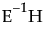. The following list describes the computation of each test statistic.
Note: After specification of a response design, the initial E and H matrices are premultiplied by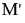and postmultiplied by M.
 Wilks’ Lambda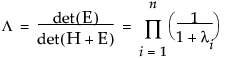Pillai’s Trace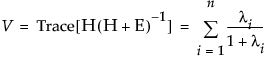Hotelling-Lawley Trace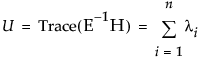Roy’s Max Root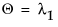, the maximum eigenvalue of.
The whole model L is a column of zeros (for the intercept) concatenated with an identity matrix having the number of rows and columns equal to the number of parameters in the model. L matrices for effects are subsets of rows from the whole model L matrix.
To compute F-values and degrees of freedom, let p be the rank of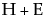. Let q be the rank of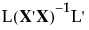, where the L matrix identifies elements of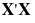associated with the effect being tested. Let v be the error degrees of freedom and s be the minimum of p and q. Also let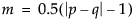and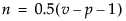.
Approximate F-statistics, gives the computation of each approximate F from the corresponding test statistic.
 Test Approximate F Numerator DF Denominator DF Wilks’ Lambda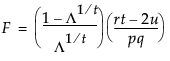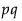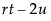Pillai’s Trace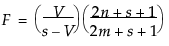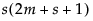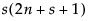Hotelling-Lawley Trace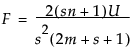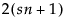Roy’s Max Root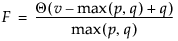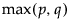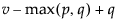where Y is the matrix of response variables, M is the response design matrix, and V is the matrix of eigenvectors offor the given test. Canonical Y’s are saved for eigenvectors corresponding to eigenvalues larger than zero.
where V is the matrix of eigenvectors of.
and the vs are columns of V, the eigenvector matrix of,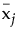refers to the multivariate least squares mean for the jth effect, g is the number of eigenvalues ofgreater than 0, and r is the rank of the X matrix.
where g is the number of eigenvalues ofgreater than 0 and the denominator L’s are from the multivariate least squares means calculations.